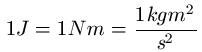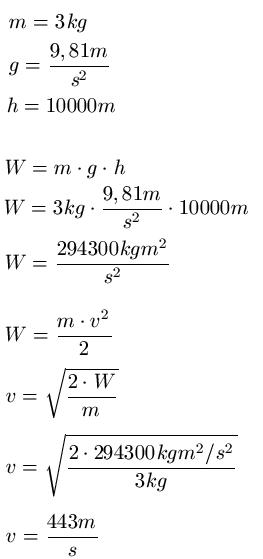# How is the mechanical energy calculated

## Mechanical energy

We will deal with mechanical energy in this article. We mainly deal with the potential and kinetic energy. This article belongs to the field of physics / mechanics.Everyone knows the term "work" from their everyday lives. You do your job, for many people that equates to eight hours sitting in an office and doing your own job. There is also a job in physics. However, this refers to how much energy it takes to push or lift an object. The work or the energy is in Joule, Newton-Meter or also in kgm2: s2 specified. The three statements express the same thing, when calculating only the use of one or the other spelling can be of interest. Again to write with:You should already know the following topics in mathematics and physics in order to subsequently understand the kinetic and potential energy.

Show:

### Potential energy

Let's come to potential energy. This is understood to be the energy that you have to apply to lift an object a certain height. Example: I lift the television 1 meter up to place it on the table. How much work / energy do I have to spend on this? The answer is the formula for potential energy.

Calculate potential energy:

• W.POT = m * g * h
• "WPOT"is the potential energy in Newton meters [Nm]
• "m" is the mass of the body being lifted in kilograms [kg]
• "g" is the acceleration due to gravity, g = 9.81m / s2 [m / s2 ]
• "h" is the height by which the object is lifted in meters [m]

If you put mass, acceleration due to gravity and altitude into the formula, you get the potential energy. If you drop the object, it gets faster and faster (because the acceleration of gravity accelerates the object). With the kinetic energy you can now calculate the speed that the object has when it hits the ground. Example: You lift a television set by 1 meter and - accidentally of course - drop it down. You have been able to calculate the potential energy with the formula from above. You use this energy in the formula for the kinetic energy and convert this formula according to the velocity v in order to calculate the impact velocity.

Show:

### Kinetic energy

The kinetic energy is calculated from the square of the speed, multiplied by the mass and divided by 2 (or multiplied by 0.5).

Calculate kinetic energy:

• W.KIN = 0.5 m v2
• W.KIN is the kinetic energy in Newton meters [Nm]
• "m" is the mass of the object in kilograms [kg]
• "v" is the speed in meters per second [m / s]

Example:
A 3kg block falls from an airplane that flies 10km above the ground. At what speed does the block hit the ground if you neglect the air resistance?

Solution: We use the formulas for potential and kinetic energy to calculate the speed. This calculation looks like this:Left:

### Who's Online

We have 301 guests online